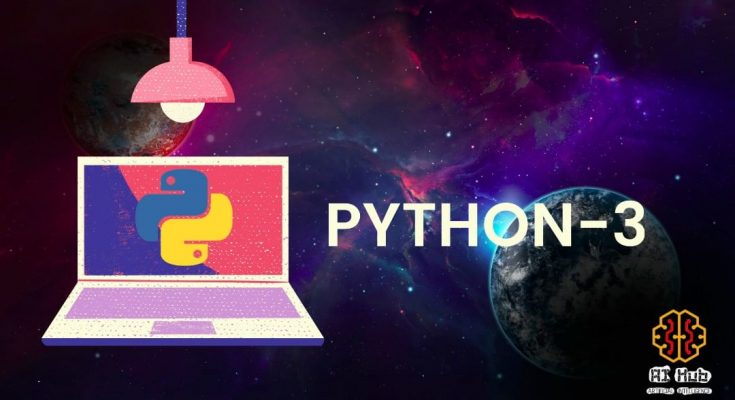# FOR LOOP IN PYTHONSuppose when we have to print “Python” 10 times, we can add ten print statements so that we can print “Python” ten times repeatedly.

1. print(‘Python’)
2. print(‘Python’)
3. print(‘Python’)
4. print(‘Python’)
5. print(‘Python’)
6. print(‘Python’)
7. print(‘Python’)
8. print(‘Python’)
9. print(‘Python’)
10. print(‘Python’)

Now, suppose if someone told you to print (‘Python’) 1000 times what will you do ? Will you execute print statement 1000 times? So to minimize such lengthy coding loops are used. We can used two kinds of loop, they are:

1. For Loop
2. While Loop
##### FOR LOOP

A FOR loop in python is used in executing blocks of code in iteration over a sequence (that is either a list, a tuple, a dictionary, a set, or a string). This operation is known as traversal. With the for loop we can execute a set of statements, once for each item in a list, tuple, set etc which is different than for loops used in other programming languages. Loop continues until we reach the last item in the sequence and the block of codes that needs to be executed in a loop is identified by indentation.

``````>>> vowel = ['a','e','i','o','u']
>>> for x in vowel:
print(x)

Output:
a
e
i
o
u``````

Here, x is the variable that takes the value of the item inside the sequence on each iteration. Each time through the loop, the variable x takes on the value of the next object in the vowel. It prints every item inside the list until it reaches the last string ‘u’. This type of loop iterates over a collection of objects, rather than specifying numeric values or conditions.

Let’s use for loop in dictionary :

``````>>> num = {'a': 1, 'b': 2, 'c': 3}
>>> for m, n in num.items():
...     print('key =', m, ', value =', n)

Output:
key = a , value = 1
key = b , value = 2
key = c , value = 3``````

In the above example, we can see that item( ) is used to access both key and value from the dictionary.

In list, set, dictionary and tuple, for loop iterates on number of items present. But in case of String ? Let’s take an example :

``````>>> a = "loop"
>>> for y in a:
print (y)

Output:
l
o
o
p``````

Since string is a single item, for loop in string slices the item into individual characters and then iterates for the number of characters present in string. As in the above example, loop iterates for four times and prints individual characters.

``````>>> b = 0
>>> a = "programming"
>>> for y in a:
b=b+1

>>> print ("\n total characters : ", b)

Output:
total characters :  11``````
##### FOR LOOP USING RANGE

To define the loop iterations for a finite number of times we use range ( ). It can also be used in list, dictionary, tuple, set, string by combining with the len( ) function to iterate through a sequence using indexing. The range( ) function returns a sequence of numbers, starting from 0 by default, and increments by 1 (by default), and ends at a specified number. But, we can also define starting value, incremental value as per our requirement.

``````>>> a = ('a','b','c','d')
>>> for item in range(len(a)):
print (a[item])

Output:
a
b
c
d``````
###### EXAMPLE 01: PRINT FIRST FIVE NUMBER
``````>>> for a in range (5):   #starts from 0 & increment by 1
print (a)

Output:
0
1
2
3
4``````
###### EXAMPLE 02: PRINT FIRST FIVE EVEN NUMBER
``````>>> for a in range (0,10,2):
print (a)

Output:
0
2
4
6
8``````

In the above example, first term is zero and in each iterations the number increments by 2 and ends at 10. In for loop, last number is ignored.

###### EXAMPLE 03: PRINT SUM OF 1 TO 100
``````>>> s= 0
>>> for b in range (1,101):
s=s+b
>>> print (" sum from 1 to 100 is : ",s)

Output:
sum from 1 to 100 is :  5050``````
##### NESTED FOR LOOPS

When a for loop is present inside another for loop then it is called a nested for loop. Lets take an example of nested for loop.

``````>>> for a in range(1,5,1):
for b in range(a):
print('*',end =" ")     #end =" " is used to print on same line
print('\n')

Output:
*

* *

* * *

* * * *``````

This page is contributed by Diwas & Sunil . If you like AIHUB and would like to contribute, you can also write an article & mail your article to  itsaihub@gmail.com . See your articles appearing on AI HUB platform and help other AI Enthusiast.🚀 I'm Diwas Pandey, a Computer Engineer with an unyielding passion for Artificial Intelligence, currently pursuing a Master's in Computer Science at Washington State University, USA. As a dedicated blogger at AIHUBPROJECTS.COM, I share insights into the cutting-edge developments in AI, and as a Freelancer, I leverage my technical expertise to craft innovative solutions. Join me in bridging the gap between technology and healthcare as we shape a brighter future together! 🌍🤖🔬

View all posts by Diwas →

### 3 Comments on “FOR LOOP IN PYTHON”

2.ปั้มไลค์ says: